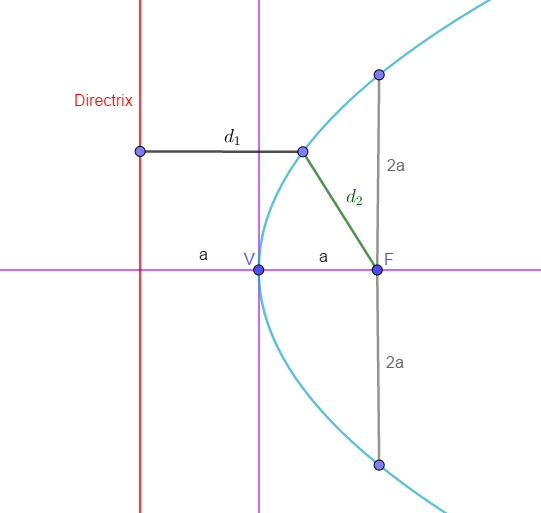# The Parabola

Parabola is a conic section. It is defined as the set of all points whose distance from a fixed point, called the focus, is equal to the distance from a fixed line, called the directrix.

The eccentricity, e of a parabola is equal to 1.Terms
Focus (F) $$a$$ distance from the vertex.
Vertex (v) $$h,k$$
Latus Rectum (LR) $$LR~=~4a$$
A line segment passing through the focus and parallel to the directrix.
$$a$$ distance between the vertex and the focus or the distance between the directix and the vertex.

#### General Equation of Parabola

$$Ax^2 + Dx + Ey + F = 0$$ or $$Cy^2 +Dx + Ey + F = 0$$

Where A, B, C, D E and F are constants.

#### Standard Equations of Parabola

Vertex at $$(0,0)$$
$$y^2 = 4ax$$ Opens Upwards
$$y^2 = -4ax$$ Opens Downwards
$$x^2 = 4ax$$ Opens to the right
$$x^2 = -4ax$$ Opens to the left
Vertex at $$(h,k)$$
$$(y-h)^2 = 4a(x-k)$$ Opens Upwards
$$(y-h)^2 = -4a(x-k)$$ Opens Downwards
$$(x-k)^2 = 4a(y-h)$$ Opens to the right
$$(x-k)^2 = -4a(y-h)$$ Opens to the left

#### How to solve $$h,k$$ and $$a$$ if the given equation is in its general form.

• Transform to standard form and get the $$h,k$$ values.
• If the general form is: $$Ax^2 + Dx + Ey + F = 0$$
• $$h = \frac{-D}{2A}$$
• $$k = \frac{D^2-4AF}{4AE}$$
• $$a = \frac{-E}{4A}$$
• If the general form is: $$Cy^2 +Dx + Ey + F = 0$$
• $$h = \frac{E^2-4CF}{4CD}$$
• $$k = \frac{-E}{2C}$$
• $$a = \frac{-D}{4C}$$